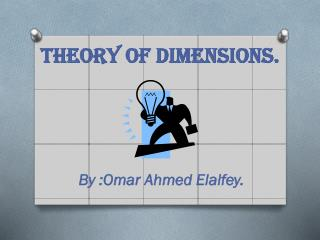# Theory of dimensions. - PowerPoint PPT PresentationDownload PresentationTheory of dimensions.

Presentation Description
Download Presentation## Theory of dimensions.

- - - - - - - - - - - - - - - - - - - - - - - - - - - E N D - - - - - - - - - - - - - - - - - - - - - - - - - - -
##### Presentation Transcript

1. Theory of dimensions. By :Omar Ahmed Elalfey.

2. Problem with Nature of Light. • Isaac Newton: Suppose light particulate his theory. • Christian Huygens: He was a strong supporter of the wave nature of light. • Max Planck: Suppose that the light travels in the form of quanta of energy. • Albert Einstein: The expansion of this theory and the imposition of the characteristics of the dual nature of light photons.

3. Double-slit Experiment.

4. Hypothesis: 1-Relative bending assumption. Deflection of moving objects in relation to each other, including so-called bow regardless of the relative measurements of the observer or any external influential force. 2-Self-bending assumption. Deflection of moving objects on the self-same degree of Anhanaúha vary according to the speed of (positive relationship), regardless of the observer measurements or any external influential force.

5. Self-bending assumption.

6. Relative bending assumption.

7. Lorentz factor.

8. Modify the Lorentz factor.

9. Time dilation equation. 1-According to theory of dimensions : 2-According to special relativity:

10. Mathematically prove of the .equation • We will express the time measured by the observer with mean

11. Squaring both sides of the previous equation, getting as the following: • Where

12. This quadratic equation of second-degree polynomial and its general formula is as follows: • With application of the equation of time zero obtained by the public image of the previous equation we get the following: • Where { express }, { express } , { express } , { express } • That means modification Lorentz's factor is • .

13. Implementation of theory of dimensions equation: • Theory dimensions Calculation • Einstein's Calculation =

14. Problems solved by Theory of dimensions. • 1-internal Geometry for movement of photon , electron (dual nature). • 2-Incident neutrino particles. • 3-Black holesparticles velocity. • 4-There speeds faster than the speed of light more than 5-fold. • 5-Solve the contradiction between the theory of relativity and quantum mechanics

15. Think special

16. Reference's. • Einstein A. (1905). ZurElectrodynamikbewegterKörper. Annal. Phys. 17, 891–921. English translation: 'On the electrodynamics of moving bodies' in The Principle of Relativity. Methuen, London (1923).  • Cassidy, David C.; Holton, Gerald James; Rutherford, Floyd James (2002). Understanding Physics. Springer-Verlag New York, Inc. ISBN0-387-98756-8, Chapter 9 §9.6, p. 422 • Cutner, Mark Leslie (2003). Astronomy, A Physical Perspective. Cambridge University Press. ISBN0-521-82196-7, Chapter 7 §7.2, p. 128 • Lerner, Lawrence S. (1996). Physics for Scientists and Engineers, Volume 2. Jones and Bertlett Publishers, Inc. ISBN0-7637-0460-1, Chapter 38 §38.4, p. 1051,1052 • Ellis, George F. R.; Williams, Ruth M. (2000). Flat and Curved Space-times, Second Edition. Oxford University Press Inc, New York. ISBN0-19-850657-0, Chapter 3 §1.3, p. 28-29